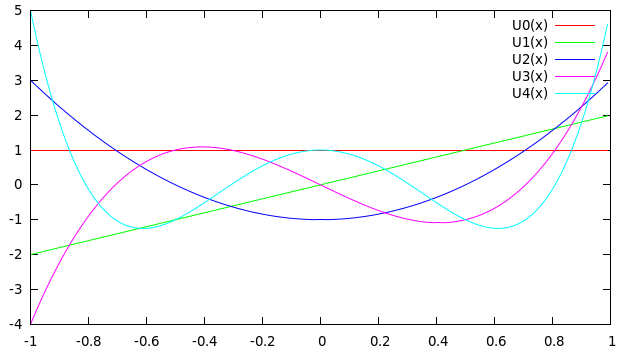# Plotting the Chebyshev Polynomials of the Second Kind using C and Gnuplot

In this post I will show you how to calculate and plot the Chebyshev Polynomials of the second kind using a C Program and Gnuplot.

We will be using the following information:$U_0(x)=1$$U_1(x)=2x$
and the recurrence relation:$U_{n+1}(x)=2xU_n(x)-U_{n-1}(x)$ where, n starts from 1.

Modifying the recurrence relation a little, so that n starts from 2, we get:$U_{n}(x)=2xU_{n-1}(x)-U_{n-2}(x)$

We will create a program that calculates the values of the Chebyshev Polynomials at various x values and for different n and store these values in a txt file. Then just plot it using Gnuplot.
We will create two functions called ‘U0’ and ‘U1’, that contain the definition of respectively.
Then we will create a function ‘Un’ that will use the first two functions and recursion to find the value of Chebyshev Polynomials for different x,n.

### PROGRAM:

```/******************************************
***********Chebyshev Polynomials***********
******************************************/
#include<stdio.h>
double U0(double x){
return 1;
}
double U1(double x){
return 2*x;
}
//General form of Chebyshev polynomial of second for a given value of n and x
double Un(double x, int n){
if(n==0){
return U0(x);
}
else if(n==1){
return U1(x);
}
else{
//using the recurrence relation
return 2*x*Un(x,n-1)-Un(x,n-2);
}
}
main(){
double x;
FILE *fp=NULL;
fp=fopen("cheby.txt","w");
//Write down the values to a file
for(x=-1;x<=1;x=x+0.01){
fprintf(fp,"%lf\t%lf\t%lf\t%lf\t%lf\t%lf\n",x,Un(x,0),Un(x,1),Un(x,2),Un(x,3),Un(x,4));
}
}
```

When you run the above C, it will generate a file called ‘cheby.txt’ which would contain 6 columns of data-points.
The first column contains the ‘x’ values and the rest of them are for$U0(x), U1(x), U2(x)...$
These can be easily plotted using Gnuplot by using the following commands:

### GnuPlot Command:

`->set xlabel 'x'`
```->plot 'cheby.txt' u 1:2 w l t "U0(x)", '' u 1:3 w l t "U1(x)", '' u 1:4 w l t "U2(x)", '' u 1:5 w l t "U3(x)", '' u 1:6 w l t "U4(x)" ```

### OUTPUT(Gnuplot):### References:[wpedon id="7041" align="center"]